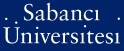# Kolmogorov problem on widths asymptotics and pluripotential theory

Yazıcı, Özcan (2008) Kolmogorov problem on widths asymptotics and pluripotential theory. [Thesis]Preview
PDF - Requires a PDF viewer such as GSview, Xpdf or Adobe Acrobat Reader
383Kb

## Abstract

Given a compact set K in an open set D on a Stein manifold Ω of dimension n, the set A[D,K] of all restriction of functions to K, analytic in D with absolute value bounded by 1 is a compact subset of C(K). The problem on the strict asymptotics for Kolmogorov diameters (widths): ln di(A[D,K]) ~ -σi[1/n],i —> ∞ was stated by Kolmogorov in an equivalent formulation for e-entropy of that set. For n = 1, this problem is solved by efforts of many authors (Erokhin, Babenko, Zahariuta, Levin-Tikhomirov, Widom, Nguyen, Skiba - Zahariuta, Fisher-Mic-cheli, et al) with σ = 1/τ where τ(K,D) := 1/2π ∫∆w (∆w is a positive measure supported on K). For n > 1 Zakharyuta conjectured that for "good" pairs (K, D) such an asymptotics holds with σ = 2π (n!/C(K,D))[1/n] where C(K,D) is the pluricapacity of the pairs (K, D) introduced by Bedford-Taylor . In [31,35] Zakharyuta reduced this problem to a problem of pluripotential theory about approximating w(K, D; z) — 1 on any compact subset of D \ K by pluricomplex Green functions on D. The latter problem which is known as Zakharyuta's conjecture has been solved by Nivoche  and Poletsky . In this thesis we give the detailed proofs of Zakharyuta's reduction of Kolmogorov problem to his conjecture and the Nivoche-Poletsky result.

Item Type: Thesis Multivariate analysis techniques. -- Potential theories. -- Hilbert spaces. -- Analytic functions. -- Çok değişkenli analiz teknikleri. -- Potansiyel teoriler. -- Hilbert uzayları. -- Analitik fonksiyonlar. Q Science > QA Mathematics 11352 IC-Cataloging 26 Feb 2009 11:45 25 Mar 2019 16:58

Repository Staff Only: item control page